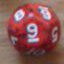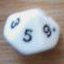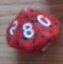Two questions on this roll definition

## Two questions on this roll definition

 Wed Feb 4 11:29:01 2015   by   Tiziano Contorno Hi Torben and all!Roll 6 "action" dice (dice have 1x "action A" face, 2x "action B" faces, 3x "action C" faces), calculate the chance to get tris of each action:POOL := 6;ACTION := choose{1, 2, 2, 3, 3, 3};ROLL := POOL# ACTION;A := count 1 <= (3 <= count (1 = ROLL));B := count 1 <= (3 <= count (2 = ROLL));C := count 1 <= (3 <= count (3 = ROLL));"A: " || 'A\"B: " || 'B\"C: " || 'CQuestions:1) When I define "ROLL", is it "rolled" once and then the resulting collection used in the following lines or is it "rolled" each time I call it (and so each collection is different)?2) How do I get statistics of "A", "B" and "C" in the same output and in 3 different lines/columns? I only managed to get each one separately :)Thank you!!! Wed Feb 4 12:55:18 2015   by   wyrdR 1) ROLL is only defined once.2) Make the last line:'("A: " |> "B: " |> "C: ")|| 'A |> 'B |> 'C Wed Feb 4 13:50:56 2015   by   Tiziano Contorno Thank you wyrdR!:D